# Last-in, first-out (LIFO) method in a periodic inventory system

Under last-in, first-out (LIFO) method, the costs are charged against revenues in reverse chronological order i.e., the last costs incurred are first costs expensed. In other words, it assumes that the merchandise sold to customers or materials issued to factory has come from the most recent purchases. The ending inventory under LIFO would, therefore, consist of the oldest costs incurred to purchase merchandise or materials inventory.

LIFO is extensively used in periodic as well as perpetual inventory system. In this article, the use of LIFO method in periodic inventory system is explained with the help of examples. To understand the use of LIFO in a perpetual inventory system, read “last-in, first-out (LIFO) method in a perpetual inventory system” article.

## Example – LIFO periodic system in a merchandising company:

A trading company has provided the following data about purchases and sales of a commodity made during the year 2016.

• Jan. 01: Beginning inventory; 1,000 units @ \$16 per unit.
• Feb. 15: Purchased; 1,800 units @ \$18 per unit.
• Apr. 15: Purchased; 1,000 units @ \$20 per unit.
• Jul. 10: Purchased; 2,000 units @ \$22 per unit.
• Oct. 20: Purchased; 1,500 units @ \$24 per unit

According to a physical count, 1,300 units were found in inventory on December 31, 2016. The company uses a periodic inventory system to account for sales and purchases of inventory.

Required: Assuming a last-in, first-out (LIFO) cost flow assumption is used, compute:

1. the cost of inventory on December 31, 2016.
2. the cost of goods sold for the year 2016.

### Solution:

#### (1). Cost of ending inventory :

Since the company is using LIFO periodic system, the 1,300 units in ending inventory would be costed using the earliest purchasing costs. The computations are given below: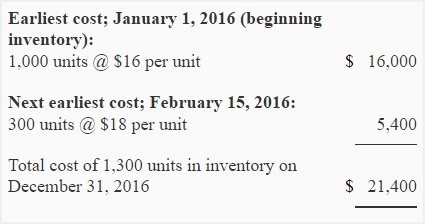#### Cost of goods sold for 2016

The cost of goods sold is equal to the cost of units sold during the year. It can be computed using one of the two methods given below:

Formula method: Under formula method, we would compute the cost of goods sold by deducting the cost of ending inventory (computed above) from the total cost of units available for sale during the period. The total cost of units available for sale is equal to cost of beginning inventory plus cost of all units purchased during the year. It can be expressed in the form of the following formulas or equations.

Cost of goods sold = Cost of units available for sale – Cost of units in ending inventory

Or

Cost of goods sold = [Cost of units in beginning inventory + Cost of units purchased during the period] – Cost of units in ending inventory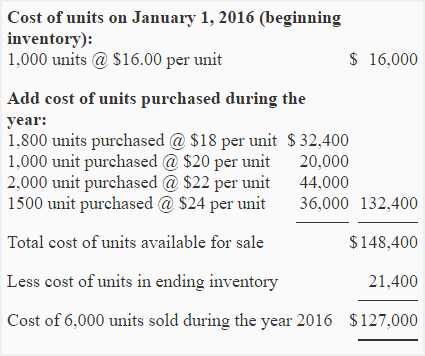Recent cost method: Under recent cost method, we would compute the total number of units sold during the year and then we would assign cost to these units using most recent costs incurred to purchase units. The computations are given below:

Number of units sold during the year = Units in beginning inventory + Units purchased during the year – Units in ending inventory

= 1,000 units + 6,300* units – 1,300 units

= 6,000 units

*1800 + 1000 + 2000 + 1500 = 6,300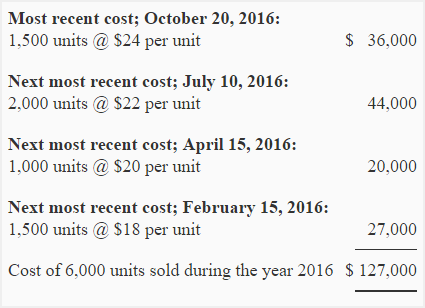LIFO periodic system is also extensively used by manufacturing companies for recording and costing materials. Consider the following example:

## Example-LIFO periodic system in a manufacturing company:

The HEC manufacturing company uses periodic inventory system. The physical inventory of materials is priced using LIFO method. The following data is available for the month of December 2016:

• Dec. 01: Beginning inventory; 50 units @ \$2.00.
• Dec. 12: Purchases; 90 units @ \$2.10.
• Dec. 19: Purchases; 230 units @ \$2.20.
• Dec. 25: Purchases; 110 units @ \$2.30.
• Dec. 29: Purchases; 40 units @ \$2.35.

On December 31, 2016, a physical count of inventory was made and 120 units of material were found in the store room.

Required:

1. Compute the total cost of inventory on December 31, 2016.
2. Compute the total cost of units issued to factory during the month of December.

### Solution:

#### (1). Cost of ending inventory:

In LIFO periodic system, the 120 units in ending inventory would be valued using earliest costs.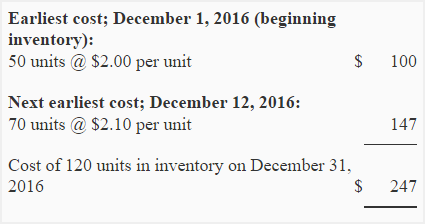#### (2). Cost of units issued to factory during December

Formula method: Under formula method, the cost of units issued to factory would be computed by deducting the cost of units in ending inventory from the total cost of units available for use during the month. The total cost of units available for use is equal to cost of units in beginning inventory plus cost of units purchased during the month.

Cost of units issued = Cost of units available for use – Cost of units in ending inventory

Or

Cost of units issued = [Cost of units in beginning inventory + Cost of units purchased during the period] – Cost of units in ending inventory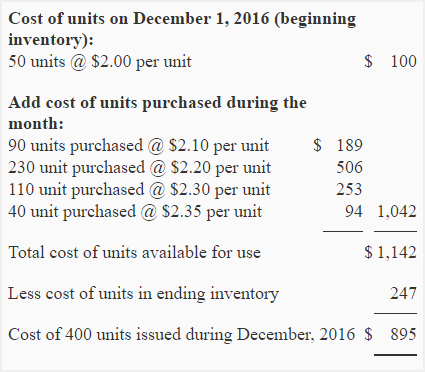Recent cost method: Under this method, the number of units issued to factory would be computed first and then they would be costed using most recent costs. In our example, the units in ending inventory would be computed and costed as follows:

Units issued to factory = Units in beginning inventory + Units purchased during the period – Units in ending inventory

= 50 units + 470* units – 120 units

= 400 units

*90 + 230 + 110 + 40 = 470Show your love for us by sharing our contents.

### 4 Comments on Last-in, first-out (LIFO) method in a periodic inventory system

1.Michael Joydher

If the question have Purchases 400 units @ 150 Taka return 150 units @ 100 Taka sales 300 units @ 250 taka and sales return 50 units @ 250 taka

How to solve by LIFO method?

2.vincent

what if you have been given some informatiom about the purchases and sales and also the sales return and purchases return,how would you account for the sales return and the purchase return when calculating the periodic fifo,lifo and the average cost

1.samrawit

thank you v.much, to help me.

3.Ngeeti naserian

Bike limited purchased 10 bikes during January and sold 6 bikes, details of which are as follows; January 1 purchased 5 bikes @\$50 each, January 5 sold 2 bikes, January 10 sold 1 bike, January 15 purchased 5 bikes @70 each, January 25 sold 3 bikes. Required; calculate FIFO and lifo